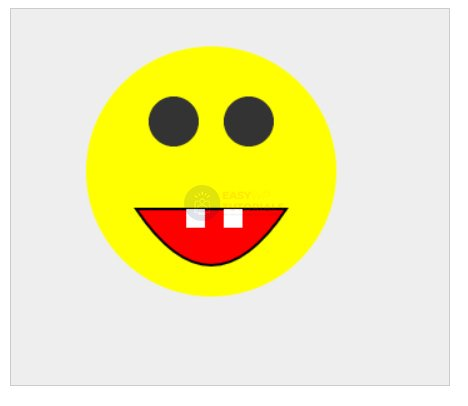# Figure Drawing In HTML5

In addition to rectangles, canvas allows you to draw more complex shapes. Complex shapes are designed using the concept of geometric paths, which represent a set of lines, circles, rectangles, and other smaller details needed to build a complex shape.

To create a new path, you need to call the beginPath() method , and after the path is completed, the closePath() method is called :

```var canvas = document.getElementById("myCanvas"),
context = canvas.getContext("2d");
context.beginPath();
context.closePath();
```

Between method calls beginPath()and closePath()there are methods that directly create different sections of the path.

### moveTo() and lineTo() methods

To start drawing a path, we need to fix the starting point of this path. This can be done with the moveTo() method , which has the following definition:

```
moveTo(x, y)
```

The method moves us to a point with x and y coordinates.

The lineTo() method draws a line. It has a similar definition:

```lineTo(x, y)
```

The method draws a line from the current position to a point with x and y coordinates.
[/js]
Now let’s draw a series of lines:

```var canvas = document.getElementById("myCanvas"),
context = canvas.getContext("2d");
context.beginPath();
context.moveTo(30, 20);
context.lineTo(100, 80);
context.lineTo(150, 30);
context.closePath();
```

Here we set the beginning of the path to the point (30, 20), then draw a line from it to the point (100, 80) and then draw another line to the point (150, 30).

Although we have drawn a few lines, until we see them, because they need to be displayed on the screen. To display the path, use the stroke() method :

```<!DOCTYPE html>
<html lang="en">

<meta charset="UTF-8">
<meta http-equiv="X-UA-Compatible" content="IE=edge">
<meta name="viewport" content="width=device-width, initial-scale=1.0">
<title>Canvas in HTML5</title>

<body>
<canvas id="myCanvas" width="400" height="200" style="background-color:rgb(205, 198, 216); border:1px solid #ccc;">
Your browser does not support Canvas
</canvas>
<script>
var canvas = document.getElementById("myCanvas"),
context = canvas.getContext("2d");
context.beginPath();
context.moveTo(30, 20);
context.lineTo(150, 140);
context.lineTo(250, 30);
context.closePath();
context.strokeStyle = "red";
context.stroke();
</script>
</body>

</html>
```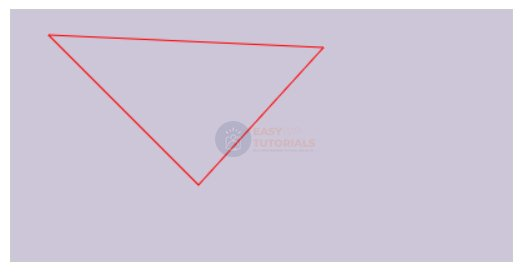Although we have drawn all two lines, in fact we will see three lines that form a triangle. The point is that the method call context.closePath() completes the path by connecting the last point to the first one. And as a result, a closed loop is formed.

If we don’t need the path closure, then we can remove the method call context.closePath():### Rect method

The rect() method creates a rectangle. It has the following definition:

```
rect(x, y, width, height)
```

Where x and y are the coordinates of the upper left corner of the rectangle relative to the canvas, and width and height are the width and height of the rectangle, respectively. Let’s draw, for example, the following rectangle:

```<!DOCTYPE html>
<html lang="en">

<meta charset="UTF-8">
<meta http-equiv="X-UA-Compatible" content="IE=edge">
<meta name="viewport" content="width=device-width, initial-scale=1.0">
<title>Canvas in HTML5</title>

<body>
<canvas id="myCanvas" width="300" height="200" style="background-color:rgb(233, 233, 255); border:1px solid #ccc;">
Your browser does not support Canvas
</canvas>
<script>
var canvas = document.getElementById("myCanvas"),
context = canvas.getContext("2d");
context.beginPath();
context.rect(30, 20, 100, 90);
context.closePath();
context.strokeStyle = "red";
context.stroke();
</script>
</body>

</html>
```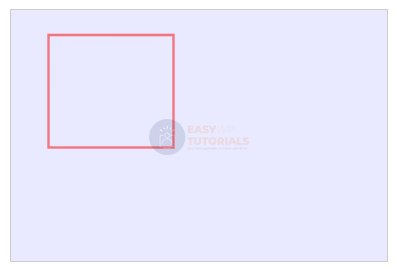It is worth noting that we could create the same rectangle from lines:

```var canvas = document.getElementById("myCanvas"),
context = canvas.getContext("2d");
context.beginPath();
context.moveTo(30, 20);
context.lineTo(130, 20);
context.lineTo(130, 110);
context.lineTo(30, 110);
context.closePath();
context.strokeStyle = "red";
context.stroke();
```

### fill() method

The fill() method fills the entire inner space of the drawn path with color:

```var canvas = document.getElementById("myCanvas"),
context = canvas.getContext("2d");
context.beginPath();
context.rect(30, 20, 100, 90);
context.closePath();
context.strokeStyle = "red";
context.fillStyle = "blue";
context.fill();
context.stroke();
```

Using the property fillStyle, again, you can set the fill color of the shape. In this case, it’s blue.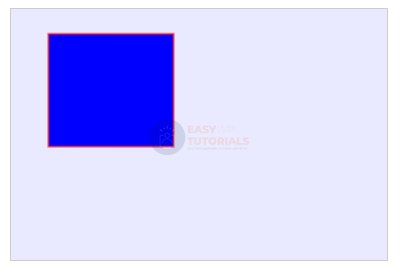### clip() method

The clip() method allows you to clip a specific area from the canvas, and anything outside that area will be ignored on subsequent rendering.

To understand this method, let’s first draw two rectangles:

```
var canvas = document.getElementById("myCanvas"),
context = canvas.getContext("2d");
// draw the first red rectangle
context.beginPath();
context.moveTo(30, 20);
context.lineTo(130, 20);
context.lineTo(130, 110);
context.lineTo(30, 110);
context.closePath();
context.strokeStyle = "red";
context.stroke();

// draw a second green rectangle
context.beginPath();
context.rect(10, 50, 180, 70);
context.closePath();
context.strokeStyle = "green";
context.stroke();
```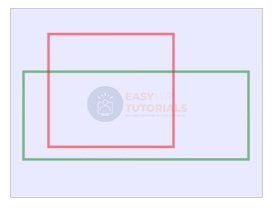Now let’s apply a method clip()to limit the drawing area to only the first rectangle:

```var canvas = document.getElementById("myCanvas"),
context = canvas.getContext("2d");
// draw the first red rectangle
context.beginPath();
context.moveTo(30, 20);
context.lineTo(130, 20);
context.lineTo(130, 110);
context.lineTo(30, 110);
context.closePath();
context.strokeStyle = "red";
context.stroke();

context.clip();

// draw a second green rectangle
context.beginPath();
context.rect(10, 50, 180, 70);
context.closePath();
context.strokeStyle = "green";
context.stroke();
```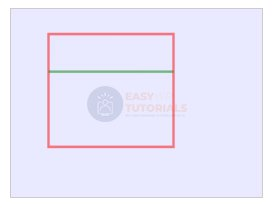Since the method call clip()comes after the first rectangle, only the part that falls into the first rectangle will be drawn from the second rectangle.

### arc() method

The arc() method adds a circle or arc to the path. It has the following definition:

```arc(x, y, radius, startAngle, endAngle, anticlockwise)
```

The following parameters are used here:

• xand y: x- and y-coordinates where the arc starts
• radius: radius of the circle around which the arch is created
• startAngleand endAngle: start and end angle that truncates the circle to the arch. The unit of measure for angles is radians. For example, a full circle is 2π radians. If, for example, we need to draw a full circle, then the endAngle parameter can be set to 2π. In JavaScript, this value can be obtained using the expression Math.PI * 2.
• anticlockwise: Direction to move around the circle while cutting off the portion of the circle bounded by the start and end angle. If value trueis counterclockwise, if value falseis clockwise.
```<!DOCTYPE html>
<html lang="en">

<meta charset="UTF-8">
<meta http-equiv="X-UA-Compatible" content="IE=edge">
<meta name="viewport" content="width=device-width, initial-scale=1.0">
<title>Canvas in HTML5</title>

<body>
<canvas id="myCanvas" width="450" height="180" style="background-color:rgb(233, 233, 255); border:1px solid #ccc;">
Your browser does not support Canvas
</canvas>
<script>
var canvas = document.getElementById("myCanvas"),
context = canvas.getContext("2d");
context.strokeStyle = "red";

context.beginPath();
context.moveTo(20, 90);
context.arc(20, 90, 50, 0, Math.PI / 2, false);
context.closePath();
context.stroke();

context.beginPath();
context.moveTo(130, 90);
context.arc(130, 90, 50, 0, Math.PI, false);
context.closePath();
context.stroke();

context.beginPath();
context.moveTo(240, 90);
context.arc(240, 90, 50, 0, Math.PI * 3 / 2, false);
context.closePath();
context.stroke();

context.beginPath();
context.arc(350, 90, 50, 0, Math.PI * 2, false);
context.closePath();
context.stroke();

</script>
</body>

</html>
```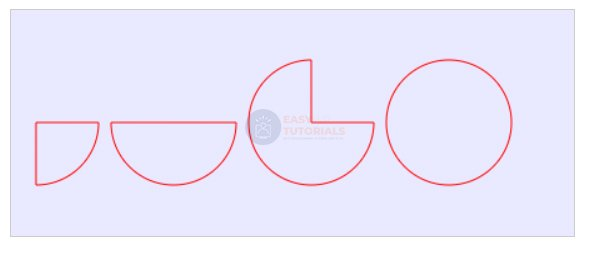The last anticlockwise parameter plays an important role, as it determines the movement in a circle, and in case of changing true to false and vice versa, we can get completely different shapes:

```
var canvas = document.getElementById("myCanvas"),
context = canvas.getContext("2d");
context.strokeStyle = "red";

context.beginPath();
context.moveTo(80, 90);
context.arc(80, 90, 50, 0, Math.PI/2, false);
context.closePath();
context.stroke();

context.beginPath();
context.moveTo(240, 90);
context.arc(240, 90, 50, 0, Math.PI/2, true);
context.closePath();
context.stroke();
```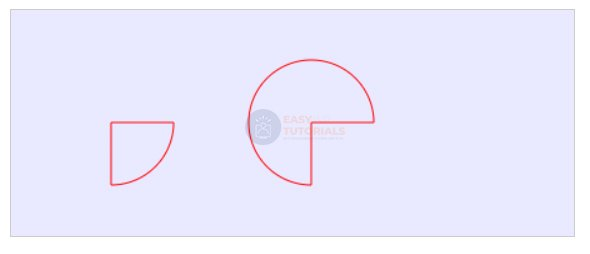### arcTo() method

The arcTo() method also draws an arc. It has the following definition:

```arcTo(x1, y1, x2, y2, radius)
```

Where x1 and y1 are the coordinates of the first control point, x2 and y2 are the coordinates of the second control point, and radius is the radius of the arc.

```var canvas = document.getElementById("myCanvas"),
context = canvas.getContext("2d");
context.strokeStyle = "red";

context.beginPath();
context.moveTo(0, 150);
context.arcTo(0, 0, 150, 0, 140)
context.closePath();
context.stroke();
```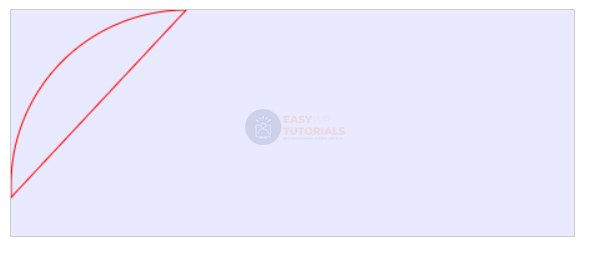Here we move first to the point (0, 150), and from this point to the first control point (0, 0) the first tangent will pass. Further from the first control point (0, 0) to the second (150, 0) the second tangent will pass. These two tangents form the arc, and 140 is the radius of the circle where the arc is truncated.

The quadraticCurveTo() method creates a quadratic curve. It has the following definition:

```quadraticCurveTo(x1, y1, x2, y2)
```

Where x1 and y1 are the coordinates of the first reference point, and x2 and y2 are the coordinates of the second reference point.

```var canvas = document.getElementById("myCanvas"),
context = canvas.getContext("2d");
context.strokeStyle = "red";

context.beginPath();
context.moveTo(20, 90);
context.closePath();
context.stroke();
```### bezierCurveTo() method. bezier curve

The bezierCurveTo() method draws a bezier curve. It has the following definition:

```bezierCurveTo(x1, y1, x2, y2, x3, y3)
```

Where x1 and y1 are the coordinates of the first control point, x2 and y2 are the coordinates of the second control point, and x3 and y3 are the coordinates of the third control point.

```var canvas = document.getElementById("myCanvas"),
context = canvas.getContext("2d");
context.strokeStyle = "red";

context.beginPath();
context.moveTo(30, 100);
context.bezierCurveTo(110, 0, 190, 200, 270, 100);
context.closePath();
context.stroke();
```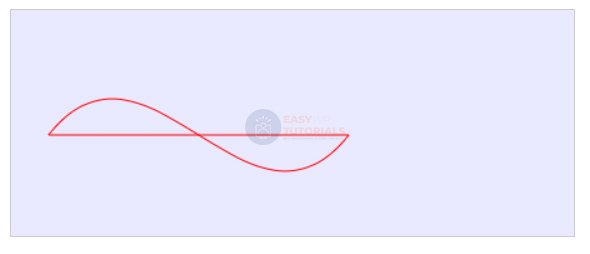### Complex figures

Let’s combine several shapes together and draw a more complex 2D scene:

```<!DOCTYPE html>
<html lang="en">

<meta charset="UTF-8">
<meta http-equiv="X-UA-Compatible" content="IE=edge">
<meta name="viewport" content="width=device-width, initial-scale=1.0">
<title>Canvas in HTML5</title>

<body>
<canvas id="myCanvas" width="350" height="300" style="background-color:#eee; border:1px solid #ccc;">
Your browser does not support Canvas
</canvas>
<script>
var canvas = document.getElementById("myCanvas");
if (canvas.getContext) {
var ctx = canvas.getContext("2d");
ctx.beginPath();
ctx.fill();
ctx.fillStyle = "yellow";
ctx.beginPath();
ctx.arc(160, 130, 100, 0, 2 * Math.PI);
ctx.fill();

ctx.beginPath();
ctx.moveTo(100, 160);
ctx.closePath();
ctx.fillStyle = "red";
ctx.fill();
ctx.lineWidth = 2;
ctx.strokeStyle = "black";
ctx.stroke();

ctx.fillStyle = "#FFFFFF";
ctx.fillRect(140, 160, 15, 15);
ctx.fillRect(170, 160, 15, 15);

ctx.beginPath();
ctx.arc(130, 90, 20, 0, 2 * Math.PI);
ctx.fillStyle = "#333333";
ctx.fill();
ctx.closePath();

ctx.beginPath();
ctx.arc(190, 90, 20, 0, 2 * Math.PI);
ctx.fillStyle = "#333333";
ctx.fill();
ctx.closePath();

}
</script>

</body>

</html>
```